Multiserver queues with general probability distributions

Textbook presentations of queueing theory start by assuming that the time between customer arrivals and the time to serve a customer are both exponentially distributed.

Maybe these assumptions are realistic enough, but maybe not. For example, maybe there’s a cutoff on service time. If someone takes too long, you tell them there’s no more you can do and send them on their merry way. Or maybe the tails on times between arrivals are longer than those of an exponential distribution.

The default model makes other simplifying assumptions than just the arrival and service distributions. It also makes other simplifying assumptions. For example, it assumes no balking: customers are infinitely patient, willing to wait as long as necessary without giving up. Obviously this isn’t realistic in general, and yet it might be adequate when wait times are short enough, depending on the application. We will remove the exponential probability distribution assumption but keep the other assumptions.

Assuming exponential gaps between arrivals and exponential service times has a big advantage: it leads to closed-form expressions for wait times and other statistics. This model is known as a M/M/s model where the two Ms stand for “Markov” and the s stands for the number of servers. If you assume a general probability distribution on arrival gaps and service times, this is known as a G/G/s model, G for “general.”

The M/M/s model isn’t just a nice tractable model. It’s also the basis of an approximation for more general models.

Let A be a random variable modeling the time between arrivals and let S be a random variable modeling service times.

The coefficient of variation of a random variable X is its standard deviation divided by its mean. Let cA and cS be the coefficients of variation for A and S respectively. Then the expected wait time for the G/G/s model is approximately proportional to the expected wait time for the corresponding M/M/s model, and the proportionality constant is given in terms of the coefficients of variation .Note that the coefficient of variation for an exponential random variable is 1 and so the approximation is exact for exponential distributions.

How good is the approximation? You could do a simulation to check.

But if you have to do a simulation, what good is the approximation? Well, for one thing, it could help you test and debug your simulation. You might first calculate outputs for the M/M/s model, then the G/G/s approximation, and see whether your simulation results are close to these numbers. If they are, that’s a good sign.

You might use the approximation above for pencil-and-paper calculations to get a ballpark idea of what your value of s needs to be before you refine it based on simulation results. (If you refine it at all. Maybe the departure of your queueing system from M/M/s is less important than other modeling considerations.)

 See Probability, Statistics, and Queueing Theory by Arnold O. Allen.

Queueing and Economies of Scale

If a single server is being pushed to the limit, adding a second server can drastically reduce wait times. This is true whether the server is a human serving food or a computer serving web pages. I first wrote about this here and I give more details here.

What if you add an extra server but you also add more customers? The second server still reduces the wait time, though clearly not by as much as if the work load didn’t increase.

If you increase the number of servers in proportion to the traffic, wait times will go down. In theory, wait times will approach zero as the traffic and number of servers go off to infinity together. So under ideal assumptions, you could lower wait times by scaling your number of customers and your number of servers. Alternatively, you could scale your number of servers but not as fast as your number of customers, and keep wait times constant while reducing payroll.

In theory, the ideal scale is as large as possible. Economies of scale are real, but so are dis-economies of scale. Eventually the latter overtakes the former. But that’s a matter for another post. For this post we’re only looking at an idealized model.

Examples

As before we assume the time between customer arrivals and the time required to serve each customer is random. (Technically, a M/M/s model.)

Assume first that the ratio of the rate at which customers arrive to the rate at which they can be served is 0.8. Here’s what happens when we increase the traffic and the number of servers proportionally.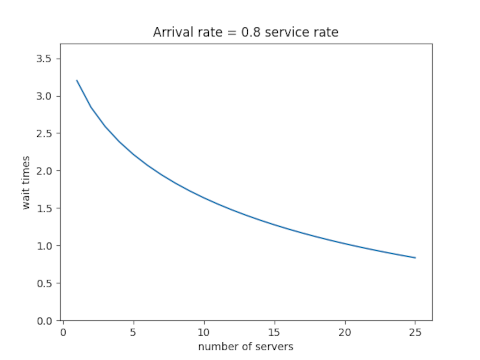Next assume that the ratio of the arrival and service rates is 0.9. Scaling the traffic and servers proportionally reduces the number of customers in line, but the wait time declines more slowly than it did when each server wasn’t so close to capacity.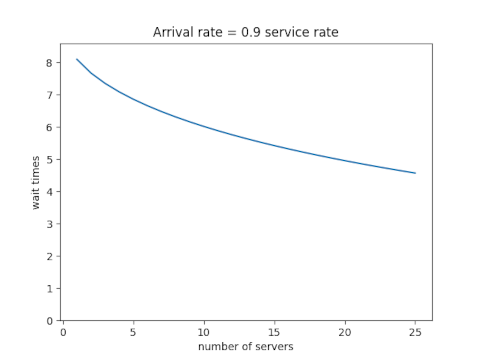Each new server helps, but each helps less than the one before. You hit diminishing return immediately.

Equations

Let ρ be the ratio of the total arrival rate to the rate at which a single server can take care of a customer and let s be the number of servers. In the examples above, ρ was 0.8s and 0.9s. The equations below don’t assume any particular relation between ρ and s except that ρ/s must be less than 1. (If ρ/s were greater than 1, the system would not approach an equilibrium; lines would grow without bound over time.)

Define p0 as follows. (NB: that’s a Roman p, not a Greek ρ. They’re visually similar, but it’s conventional notation in queueing theory.)Then the expected wait time isBy the way, we’ve assumed the time between arrivals and the time to serve a customer are both exponentially distributed. What if they’re not? That is the subject of my next post.

Swanson’s rule of thumb

Swanson’s rule of thumb  says that the mean of a moderately skewed probability distribution can be approximated by the weighted average of the 10th, 50th, and 90th percentile, with weights 0.3, 0.4, and 0.3 respectively. Because it is based on percentiles, the rule is robust to outliers. Swanson’s rule is used in the oil and gas industry, but I don’t believe it’s widely known outside of that sector.

This post will apply Swanson’s rule to three distributions to see how well it does. I’ll plot the bias in Swanson’s rule and skewness for three distributions families as the shape parameters vary.

First, here’s a plot for the gamma distribution with shape parameter k varying from 0.5 to 10.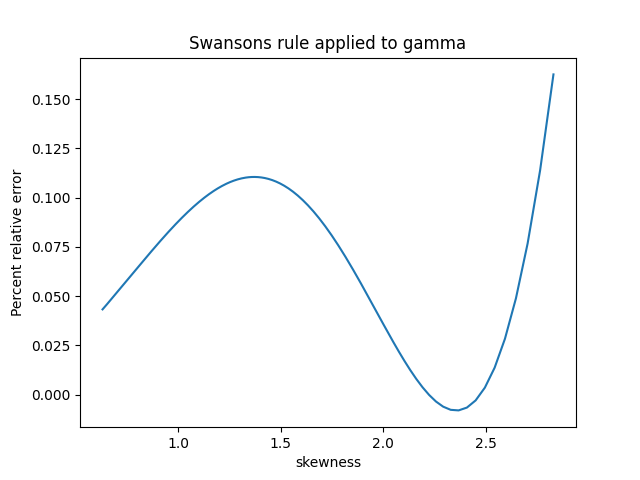The skewness of a gamma distribution is 1/√k. So unless k is close to 0, the skewness of a gamma distribution is small and the error in Swanson’s rule is very small.

For the log normal I let the shape parameter σ vary from 0.01 to 1; I didn’t use larger parameters because skewness for the log normal increases very rapidly as a function of σ. Over the range I plotted the percentage relative error in Swanson’s rule is roughly equal to the skewness.The skewness of a Pareto distribution is undefined unless the shape parameter is greater than 3. The plot below is based on shape parameters between 3.1 and 4. The skewness decreases as the shape parameter increases, asymptotically approaching 2.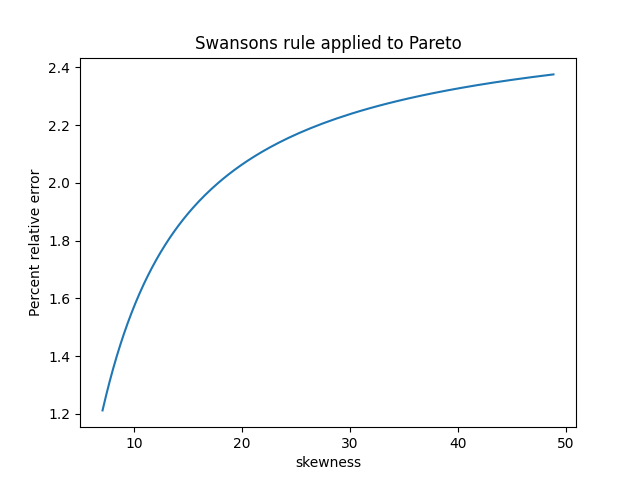Based on these examples, Swanson’s rule does indeed work pretty well when skewness is small. It works well for the gamma distribution in general because skewness is always small (unless the shape parameter k is tiny). But the log normal distribution shows that the assumption of small skewness is important.

For the Pareto distribution, Swanson’s rule works well when the shape parameter is large and the skewness is small. But this was kind of a stress test for Swanson’s rule because often the Pareto distribution is used when the skewness is large if not infinite.

 Swanson’s 30-40-30 rule. A. Hurst, G. C. Brown, R. I. Swanson. AAPG Bulletin, December 2000

Random sampling to save money

I was stunned when my client said that a database query that I asked them to run would cost the company \$100,000 per year. I had framed my question in the most natural way, not thinking that at the company’s scale it would be worth spending some time thinking about the query.

Things have somewhat come full circle. When computers were new, computer time was expensive and programmer time was dirt cheap by comparison. Someone might be scolded for using computer time to do what a programmer could do. Now of course programmer time is expensive and computer time is cheap. Usually.

But when dealing with gargantuan data sets, the cost of computer time might matter a great deal, especially when renting services from a cloud provider. Maybe you can find a more clever way to run an enormous query. Or even better, maybe you can find a way to avoid running an enormous query.

One way to save time and money is to base decisions on random samples rather than exhaustive queries. This requires a little preparation. How big a sample do you need? How exactly are you going to take a sample? What kind of uncertainty do you have in your result when you’re done? You can afford to think about these questions a long time if it saves tens of thousands of dollars per year.

Aesthetic uses of Latin squares

We think they like randomness in design, but we don’t exactly. People like things that are sorta random, but not too random. When you literally scatter things randomly, they looked too clumped .

There are many ways around this problem, variations on randomness that people find more aesthetically pleasing. One of these ways is random Latin squares.

A Latin square of size n is an n × n grid filled with n different things—numbers, letters, colors, etc.— such that each kind of thing appears exactly once in each row and in each column. For example, 26 × 26 grid filled with the letters of the English alphabet is a Latin square if every row and every column contains the entire alphabet; no letters repeated and no letters missing.

A random Latin square is an arrangement that is random but subject to the constraint that it be a Latin square. Random Latin squares look nice because they’re somewhat random but they avoid certain kinds of repetition.

Suppose you have five different colors of tile and you need to lay down the tiles in a 10 × 20 grid. You want to lay down the tiles “randomly” but you don’t want too many of any one color to appear in a small area.

One way to do this would be to divide the 10 × 20 space into a grid of grids. Each subgrid is a 5 × 5 Latin square. Here’s an example.The image above is a 2 × 4 grid of 5 × 5 grids, and each of the 5 × 5 grids is a Latin square chosen at random.

Here’s another example with five different colors and different random Latin squares.If you look carefully, you’ll spot a few places where tiles of the same color touch on an edge. This cannot happen within a Latin square, but it can happen where two different Latin squares are next to each other. So the randomization scheme described here allows for a few tiles of the same color to touch but not many.

I expect randomized Latin squares are used this way fairly often. You’d never know, and that’s kinda the point. Someone would have to stare at a pattern like this a long time before they realized that horizontal or vertical segments of five do not repeat a color.

Related posts

 One way to detect fraud is to look for fake randomness. When people try to make something look random, they usually fail and leave statistically detectable fingerprints.

Yule statistics Y and Q

I recently wrote about the Yule-Simon distribution. The same Yule, George Udny Yule, is also known for the statistics Yule’s Y and Yule’s Q. The former is also known as the coefficient of colligation, and the latter is also known as the Yule coefficient of association.

Both measure how things are related. Given a 2 × 2 contingency tablewith non-negative entries, Yule’s Y is defined byand Yule’s Q is defined byBoth essentially measure how much bigger ad is than bc but are weighted differently.

The algebraic properties of these two statistics may be more interesting than their statistical properties. For starters, both Y and Q produce values in the interval (-1, 1), and each is an inverse of the other:There’s some simple but interesting algebra going on here. DefineThis simple function comes up surprisingly often. It’s a Möbius transformation, and it comes up in diverse applications such as designing antennas and mental math shortcuts. More on that here.

If we defineandthen W generalizes both Q and Y: setting p = 1 gives us Q and setting p = 1/2 gives us Y.

Given the value of W with subscript p, we could easily solve for the value of W with another subscript q, analogous to solving Q for Y and Y for Q above.If you’re expecting f-1 rather than f over the first arrow, you’re right, but f is its own inverse so we could just write f instead.

Comparing three discrete power laws

Yesterday I wrote about the zeta distribution and the Yule-Simon distribution and said that they, along with the Zipf distribution, are all discrete power laws. This post fills in detail for that statement.

The probability mass functions for the zeta, Zipf, and Yule-Simon distributions are as follows.Here the subscripts ζ, y, and z stand for zeta, Yule, and Zipf respectively. The distribution parameters follow after the semicolon.

Comparing Zipf and zeta

The Zipf distribution is only defined on the first N positive integers. The normalizing constant for the Zipf distribution is the Nth generalized harmonic number with exponent s.As N goes to infinity, HN,s converges to ζ(s); this is the definition of the ζ function. So the Zipf and zeta distributions are asymptotically equal.

Comparing zeta and Yule

This post showed that for large k,That is, fy(k, ρ) is proportional to a power of k, except k is shifted by an amount ρ.

To compare the zeta and Yule distributions we’ll need to compare zeta with s+1 to Yule with ρ in order to make the exponents agree, and we’ll need to shift the zeta distribution by s+1.

When we plot the ratio of the pmfs, we see that the distributions agree in the limit as k gets large.In this plot s = ρ = 1.5.

The ratio is approaching a constant, and in fact the limit isbased on the ratio of the proportionality constants.

Comment

Note that the three distributions are asymptotically proportional, given the necessary shift in k, but in different ways. The Zipf distribution converges to the zeta distribution, for every k, as N goes to infinity. The zeta and Yule distributions are asymptotically proportional as k goes to infinity. So one proportion is asymptotic in a parameter N and one is asymptotic in an argument k.

The zeta distribution

The previous post on the Yule-Simon distribution mentioned the zeta distribution at the end. This is a powerlaw distribution on the positive integers with normalizing constant given by the Riemann zeta distribution. That is, the zeta distribution has density

f(k; s) = ks / ζ(s).

where k is a positive integer and s > 1 is a fixed parameter.

For s > 1, the zeta function is defined as the sum of the positive integers to the power negative s, so ζ(s) is essentially defined as the normalizing constant of the zeta distribution.

I wanted to make a couple comments about this. First, it shows that the zeta function appears in applications outside of number theory. Second, when working with the zeta distribution, it would be useful to have an estimate for ζ(s).

Zeta function bounds

The integral test in calculus is typically presented as a way to test whether an infinite sum converges. This is a shame. In analysis as in statistics, estimation is better than testing. Testing throws away continuous information and replaces it with a single bit.

Let f(x) be a decreasing function; we have in mind f(x) = 1/xs. Then for any n,To estimate a sum over k, we sum the first n terms directly and apply the inequalities above for the rest. Typically n will be small, maybe 0 or 1. A larger value of n will give more accurate bounds but at the expense of a more complicated expression.

When f(x) = 1/xs and n is at least 1, we haveThis saysThis gives a good lower bound but a bad upper bound when we choose n = 1.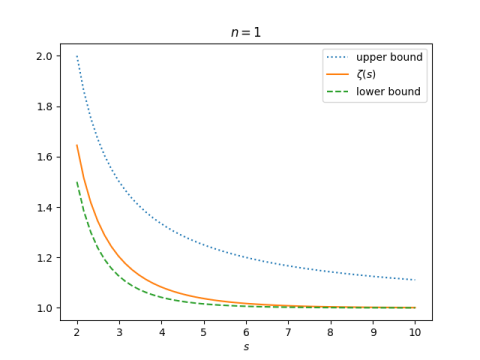But it gives a much better upper bound when we choose n = 2.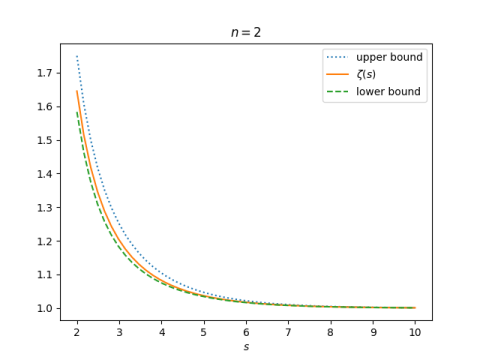Yule-Simon distribution

The Yule-Simon distribution, named after Udny Yule and Herbert Simon, is a discrete probability with pmfThe semicolon in f(k; ρ) suggests that we think of f as a function of k, with a fixed parameter ρ. The way the distribution shows the connection to the beta function, but for our purposes it will be helpful to expand this function usingand soIgnore the first part of the last line, ρ Γ(ρ + 1), because it doesn’t involve k. It helps to ignore proportionality constants in probability densities when they’re not necessary. What’s left is the (ρ + 1) falling power of k + ρ.

For large values of k, the falling power term is asymptotically equal to kρ+1. To see this, let k = 1000 and ρ = 3. Then we’re saying that the ratio of

1003 × 1002 × 1001 × 1000

to

1000 × 1000 × 1000 × 1000

is approximately 1, and the ratio converges 1 as k increases.

This says that the Yule-Simon distribution is a power law in the tails, just like the Zipf distribution and the zeta distribution. Details of the comparison between these three distributions are given here.

Probability of a magical permutation

Take a permutation of the numbers 1 through n² and lay out the elements of the permutation in a square. We will call a permutation a magic permutation if the corresponding square is a magic square. What is the probability that a permutation is a magic permutation? That is, if you fill a grid randomly with the numbers 1 through n², how likely are you to get a magic square?

For example, we could generate a random permutation in Python and see whether it forms a magic square.

>>> import numpy as np
>>> np.random.permutation(9).reshape(3,3)
array([[4, 7, 3],
[2, 6, 5],
[8, 0, 1]])

The first row adds up to 14 and the second row adds up to 13, so this isn’t a magic square. We could write a script to do this over and over and check whether the result is a magic square.

The exact number of magic squares of size n is known for n up to 5, and we have Monte Carlo estimates for larger values of n.

The number of unique magic squares, modulo rotations and reflections, of size 1 through 5 is

1, 0, 1, 880, 275305224.

For n > 2 the total number of magic squares, counting rotations and reflections as different squares, is 8 times larger than the numbers above. This is because the group of rotations and reflections of a square, D4, has 8 elements.

The probability that a permutation of the numbers 1 through 9, arranged in a square, gives a magic square is 8/9!. The corresponding probability for the numbers 1 through 16 is 8 × 880/16!, and for the numbers 1 through 25 we have 8 × 275305224/25!.

|---+-------------|
| n | Prob(magic) |
|---+-------------|
| 3 | 2.20459e-05 |
| 4 | 3.36475e-10 |
| 5 | 1.41990e-16 |
|---+-------------|

It looks like the exponents are in roughly a linear progression, so maybe you could fit a line fairly well to the points on a logarithmic scale. In fact, empirical studies suggest that the probability that a permutation of the first n² positive integers is magic decreases a little faster than exponentially.

We could fit a linear regression to the logs of the numbers above to come up with an estimate for the result for n = 6. We expect the estimate could be pretty good, and likely an upper bound on the correct answer. Let’s see what actually happens with a little R code.

> x <- c(3,4,5)
> y <- 8*c(1/factorial(9), 880/factorial(16), 275305224/factorial(25))
> m <- lm(log(y) ~ x)
> predict(m, data.frame(x=c(6)))
1
-48.77694

This says we’d estimate that the natural log of the probability that a permutation of the first 6² positive integers is magic is -48.77694. So the estimate of the probability itself is

exp(-48.77694) = 6.55306 × 10-22

We don’t know the number of magic squares of size n = 6, but the number of distinct squares has been estimated  at

(0.17745 ± 0.00016) × 1020

and so the total number including rotations and reflections would be 8 times higher. This says we’d expect our probability to be around

8 × 0.17745 × 1020 / 36! = 3.18162 × 10-22

So our estimate is off by about a factor of 2, and as predicted it does give an upper bound.

Looking back at our regression model, the slope is -12.88 and the intercept is 28.53. This suggests that an upper bound of the probability of a permutation of size n² being magical is

exp(28.53 – 12.88 n).

In closing I’d like to point out that the estimate for n = 6 that we’re referencing above did not come from simply permuting 36 integers over and over and counting how many of the permutations correspond to magic squares. That would take far too long. It’s quite likely that after the first billion billion tries you would not have seen a magic permutation. To estimate such a small number to four significant figures requires a more sophisticated Monte Carlo procedure.

Related posts

 See OEIS sequence A006052